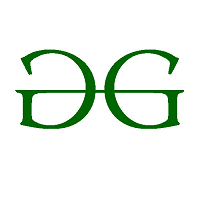# Box Blur Algorithm – With Python implementation

The pixels in an image are represented as integers. After blurring each pixel ‘x’ of the resulting image has a value equal to the average of the pixels surrounding ‘x’ including ‘x’. For example, consider a 3 * 3 image asThen, the resulting image after blur is blurred_image =So, the pixel of blurred image is calculated as (1 + 1 + 1 + 1 + 7 + 1 + 1 + 1 + 1) / 9 = 1.66666 = 1

## Box Blur Algorithm –

Box blur is also known as box linear filter. Box blurs are frequently used to approximate Gaussian blur.

A box blur is generally implemented as an image effect that affects the whole screen. The blurred colour of the current pixel is the average of the current pixel’s colour and its 8 neighbouring pixels.

Note: For each 3 * 3 pixel matrix there is one blurred pixel calculated as above.

FOr Example, Consider the below image.It’s blurred image is given below:Explanation:
There are four 3 * 3 matrix possible in the above image. So there are 4 blurred pixel in the resulting image. The four matrices are:,,,
andImplementation in Python:

 def square_matrix(square):      """ This function will calculate the value x          (i.e. blurred pixel value) for each 3 * 3 blur image.      """     tot_sum = 0            # Calculate sum of all the pixels in 3 * 3 matrix      for i in range(3):          for j in range(3):              tot_sum += square[i][j]                     return tot_sum // 9     # return the average of the sum of pixels     def boxBlur(image):      """      This function will calculate the blurred       image for given n * n image.       """     square = []     # This will store the 3 * 3 matrix                    # which will be used to find its blurred pixel                          square_row = [] # This will store one row of a 3 * 3 matrix and                       # will be appended in square                             blur_row = []   # Here we will store the resulting blurred                      # pixels possible in one row                       # and will append this in the blur_img             blur_img = [] # This is the resulting blurred image             # number of rows in the given image      n_rows = len(image)              # number of columns in the given image      n_col = len(image)              # rp is row pointer and cp is column pointer      rp, cp = 0, 0             # This while loop will be used to       # calculate all the blurred pixel in the first row       while rp <= n_rows - 3:           while cp <= n_col-3:                             for i in range(rp, rp + 3):                                     for j in range(cp, cp + 3):                                             # append all the pixels in a row of 3 * 3 matrix                      square_row.append(image[i][j])                                         # append the row in the square i.e. 3 * 3 matrix                   square.append(square_row)                  square_row = []                             # calculate the blurred pixel for given 3 * 3 matrix               # i.e. square and append it in blur_row              blur_row.append(square_matrix(square))              square = []                             # increase the column pointer              cp = cp + 1                    # append the blur_row in blur_image          blur_img.append(blur_row)          blur_row = []          rp = rp + 1 # increase row pointer          cp = 0 # start column pointer from 0 again             # Return the resulting pixel matrix      return blur_img     # Driver code  image = [[7, 4, 0, 1],           [5, 6, 2, 2],           [6, 10, 7, 8],           [1, 4, 2, 0]]             print(boxBlur(image))

Output:

[[5, 4],
[4, 4]]


Test case 2:

 image = [[36, 0, 18, 9],            [27, 54, 9, 0],            [81, 63, 72, 45]]     print(boxBlur(image))

Output:

[[40, 30]]

Further Read: Box Blur using PIL library | Python

My Personal Notes arrow_drop_upCheck out this Author's contributed articles.

If you like GeeksforGeeks and would like to contribute, you can also write an article using contribute.geeksforgeeks.org or mail your article to contribute@geeksforgeeks.org. See your article appearing on the GeeksforGeeks main page and help other Geeks.

Please Improve this article if you find anything incorrect by clicking on the "Improve Article" button below.

Article Tags :

Be the First to upvote.

Please write to us at contribute@geeksforgeeks.org to report any issue with the above content.